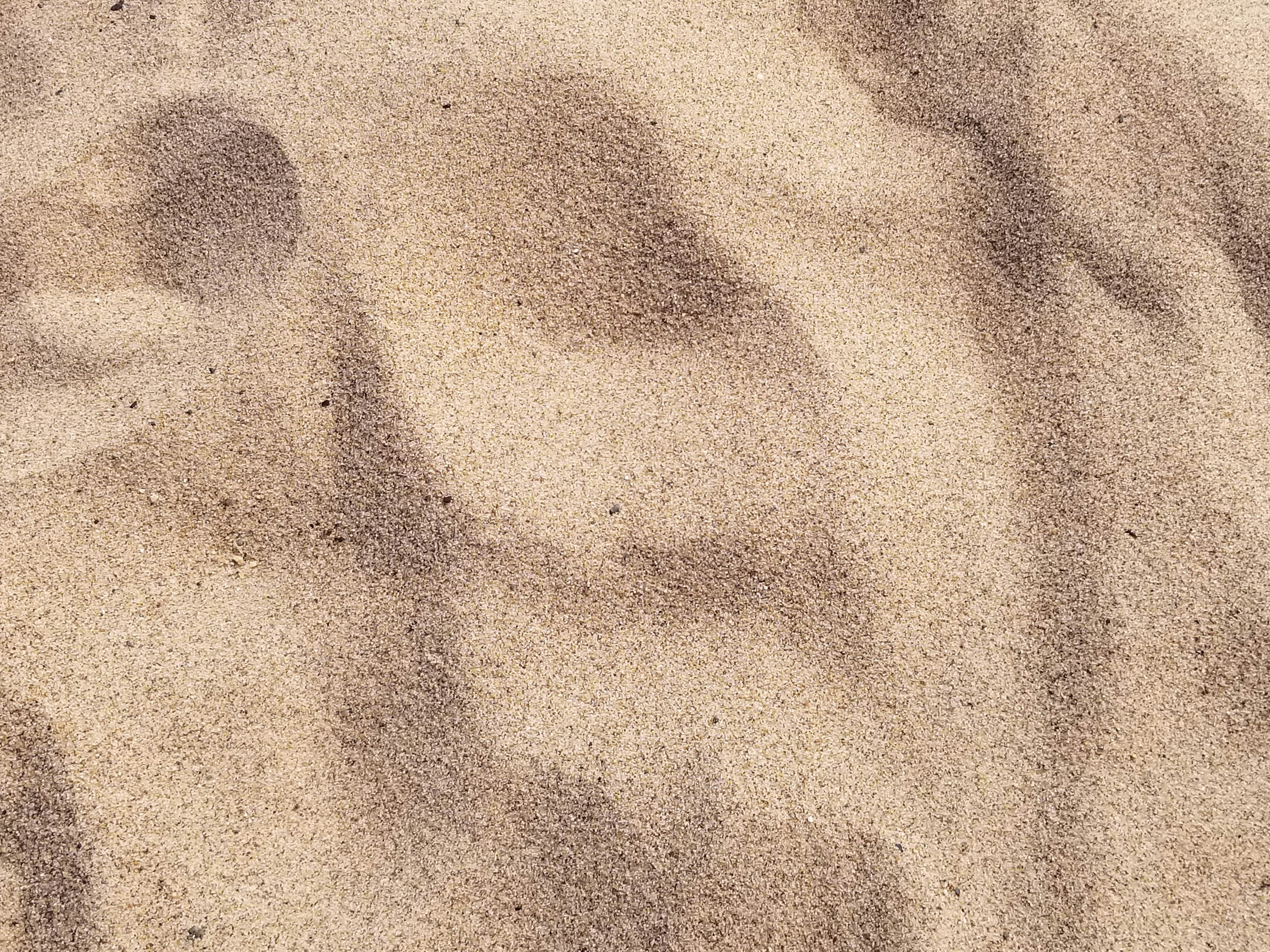Template Price Variations
Share
Explore#DB SandDB Sand

Example of volume based pricing on the canvas
\$ 0
(price As Of)
DB Sand
1
Name
Product
Price
VolumeInKg
pricePerKg
1
1 | Sand \$176.74 per 1,000 kg
Sand
\$176.74
1,000
\$0.177
2
2 | Sand \$1,060.00 per 10,000 kg
Sand
\$1,060.00
10,000
\$0.106
3
3 | Sand \$3,990.00 per 50,000 kg
Sand
\$3,990.00
50,000
\$0.080
4
4 | Sand \$5,000.00 per 70,000 kg
Sand
\$5,000.00
70,000
\$0.071
5
5 | Sand \$6,900.00 per 100,000 kg
Sand
\$6,900.00
100,000
\$0.069
There are no rows in this table
You test this best with a number like
[ ]
In this example we work with the Price As Of, see my blog to understand this principle.
The additional complexity in this example is that you cannot buy any volume, it should always be at least 1000 kg and this is because this is the lowest value in the range. Meaning that if you want to buy 100, you buy 1000 and if you want to buy 1100 you have to purchase 2000.
Below the first steps:
@1 | Sand \$176.74 per 1,000 kg
@1 | Sand \$176.74 per 1,000 kg
\$0.177
0.17674

### CODE part A

→ part 01: we ask for all values when they are lower or equal to the volumeInkg. This results in a list of items that fit this specific criteria.
`[DB Sand].Filter( VolumeInKg <= _Volume.IfBlank([DB Sand].VolumeInKg.Min()))`
→ part 02: since we asked for a list of items, but we only need one, we added Last() and not First() and this due to the smaller or equal to logic.
`[DB Sand].Filter( VolumeInKg <= _Volume.IfBlank([DB Sand].VolumeInKg.Min())).Last()`
→ part 03: so far we asked for nothing special and then the fall back value is what you see in the display column. It is time to ask for the price per kg and so we add this parameter, but not before we renamed the main part of the formula as 'Base'. Renaming is an easy way to shorten your functions.
`[DB Sand].Filter( VolumeInKg <= _Volume.IfBlank([DB Sand].VolumeInKg.Min())).Last().WithName(Base,`` `` Base.pricePerKg)`
→ part 04: to get the price we multiply with the volume using the function Product()
`[DB Sand].Filter( VolumeInKg <= _Volume.IfBlank([DB Sand].VolumeInKg.Min())).Last().WithName(Base,`` ``Product(Base.pricePerKg,_Volume))`
So far we did not take into account that the volume bought should be a multiplier of the lowest volume in the list, in our case 1000. We need in part B we bring the multiplier into account.

### CODE part B

The first part of solution for the rounding problem is the one below:
[ ]
`Quotient( _Volume , [DB Sand].VolumeInKg.Min()).RoundUp()`
The second part is bringing the value back
0
`Quotient( _Volume , [DB Sand].VolumeInKg.Min()).RoundUp() * [DB Sand].VolumeInKg.Min()`
Last we relate his volume to the price per unit.
0
and we do so by integrating step 4 of part A with the previous step.
`[DB Sand].Filter( VolumeInKg <= _Volume.IfBlank([DB Sand].VolumeInKg.Min())).Last().WithName(Base,`` `` Quotient( _Volume , [DB Sand].VolumeInKg.Min()).RoundUp() * [DB Sand].VolumeInKg.Min().WithName(NewVolume, `` ``Product(Base.pricePerKg,newVolume)))`
to get a proper formatting we wrap the outcome in a function that ‘formats’ the output:
`[DB Sand].Filter( VolumeInKg <= _Volume.IfBlank([DB Sand].VolumeInKg.Min())).Last().WithName(Base,`` `` Quotient( _Volume , [DB Sand].VolumeInKg.Min()).RoundUp() * [DB Sand].VolumeInKg.Min().WithName(NewVolume, `` ``Product(Base.pricePerKg,newVolume))).WithName([Amount to pay],`` `` Format("\$ {1}",[Amount to pay])`
` )`